# Sparse Gaussian MRF Mixtures for Anomaly Detection

## 1 Overview

The sGMRFmix package provides an implementation of the algorithm presented by Ide et al. (2016). It is a novel anomaly detection method for multivariate noisy sensor data. It can automatically handle multiple operational modes. And it can also compute variable-wise anomaly scores.

First, we prepare a multivariate training data that contains no anomaly observations. Here we generate a data that consists of four parts and repeats two operational modes.

``````library(sGMRFmix)
set.seed(314)
train_data <- generate_train_data()
plot_multivariate_data(train_data)``````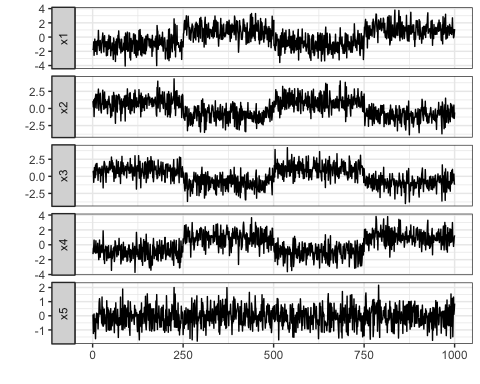Second, we prepare a multivariate test data that contains some anomaly values. Here we generate a data that consists of 500 normal observations and 500 anomaly observations. The normal part in the test data consists of two operational modes that also have seen in the training data. Note that the variable `x5` has no difference in the normal and anomaly part.

``````test_data <- generate_test_data()
plot_multivariate_data(test_data)``````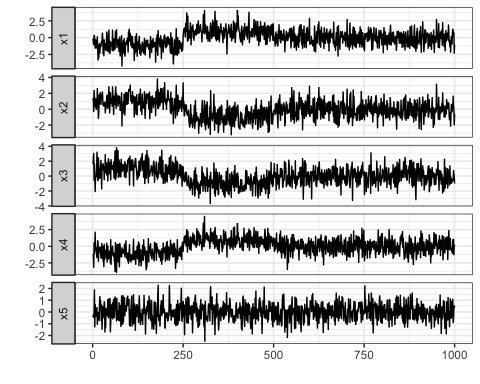We input the training data to the `sGMRFmix()` function to learn the two operational modes. Then we compute variable-wise anomaly scores for test data using the result.

``````fit <- sGMRFmix(train_data, K = 7, rho = 0.8, verbose = FALSE)
anomaly_score <- compute_anomaly_score(fit, test_data)
plot_multivariate_data(anomaly_score, fix_scale = TRUE) + ylim(NA, 50)``````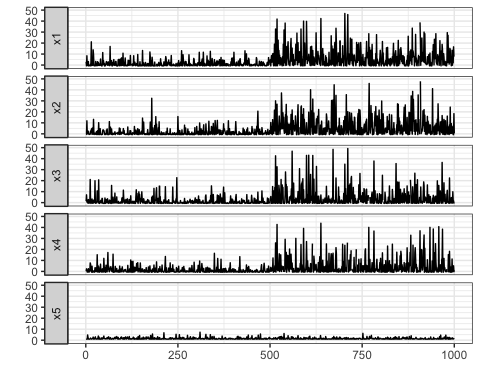You can see that high anomaly scores appear in the latter part of the test data. And you can also see the variable `x5` has no high anomaly scores.

## 2 Installation

You can install the sGMRFmix package from CRAN.

``install.packages("sGMRFmix")``

You can also install the package from GitHub.

``````install.packages("devtools") # if you have not installed "devtools" package
devtools::install_github("hoxo-m/sGMRFmix")``````

The source code for sGMRFmix package is available on GitHub at

## 3 Details

### 3.1 Basics

The sGMRFmix package mainly provides two functions as follows:

• `sGMRFmix` to fit the model and
• `compute_anomaly_score` to compute anomaly scores.
``````library(sGMRFmix)

fit <- sGMRFmix(train_data, K, rho)
anomaly_score <- compute_anomaly_score(fit, test_data)``````

There are two hyperparameters as below.

• `K` is a max number of mixture components.
• `rho` is a constant that multiplies to the penalty term in the model.

You only need to set `K` a large enough number because the algorithm identifies major dependency patterns from the data via the sparse mixture model.

On the other hand, you should determine `rho` an optimal value to maximize the performance of anomaly detection.

### 3.2 Data

To fit the model, you must prepare two kinds of data as follows:

• Training data without anomalies to fit the model and
• Labeled test data that contains anomalies to tuning the hyperparameter `rho`.

The package provides several functions to generate synthetic data.

``````set.seed(314)
train_data <- generate_train_data()
head(train_data)
#>           x1         x2          x3         x4          x5
#> 1 -1.8269027  1.0745443  0.56970302 -1.2568921  0.08997801
#> 2 -1.6120444  0.2252822  0.74793059 -0.4788593 -1.25022520
#> 3 -0.6661666  1.2513592 -0.87397154 -0.3561145 -0.59305259
#> 4 -2.5561030 -1.1858031  0.08327536  0.6284850  0.12603220
#> 5 -1.3413068  0.7235066  1.28744741 -0.9630511 -1.10169444
#> 6 -0.2811875  1.4805043  0.33644955 -0.5148741 -0.20605220
test_data <- generate_test_data()
head(test_data)
#>           x1         x2          x3          x4          x5
#> 1 -0.1534335  1.1129516  1.61478168 -1.15048817 -0.42104579
#> 2 -0.6737145  1.3716102  2.30689870 -1.41350416 -0.26243160
#> 3 -1.0491129 -0.2908006  3.04536973 -3.15425980 -0.46513038
#> 4  0.3412411  2.3378319 -0.56224407  0.51954178  0.05482343
#> 5  0.2378417  1.6324559  1.63852619 -1.11463852  1.87995270
#> 6 -0.3792480  0.3993461 -0.03158052  0.06132161 -1.27252108
test_labels <- generate_test_labels()
head(test_labels)
#>      x1    x2    x3    x4    x5
#> 1 FALSE FALSE FALSE FALSE FALSE
#> 2 FALSE FALSE FALSE FALSE FALSE
#> 3 FALSE FALSE FALSE FALSE FALSE
#> 4 FALSE FALSE FALSE FALSE FALSE
#> 5 FALSE FALSE FALSE FALSE FALSE
#> 6 FALSE FALSE FALSE FALSE FALSE``````

Also, the package provides a function to visualize these data.

``plot_multivariate_data(train_data)````plot_multivariate_data(test_data, label = test_labels)``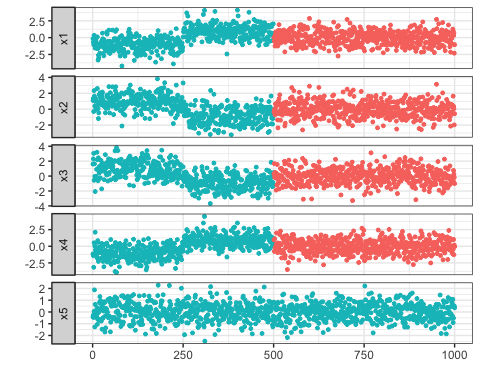### 3.3 Fitting Model

The package provides a function `sGMRFmix()` to fit the model named Sparse Gaussian Markov Random Field Mixtures (Ide et al., 2016). It can automatically handle multiple operational modes and allows to compute variable-wise anomaly scores.

``````fit <- sGMRFmix(train_data, K = 7, rho = 0.8, verbose = FALSE)
fit``````
``````#>
#> Call:
#> sGMRFmix(x = train_data, K = 7, rho = 0.8, verbose = FALSE)
#>
#> Data: 1000 x 5
#> Parameters:
#>   K:    7
#>   rho:  0.8
#> Estimated:
#>   Kest: 2
#>   pi:   0.502 0.498
#>   m, A, theta, H, mode``````

As the result of we set `K` to 7 and fit the model, the number of mixtures `Kest = 2` has been obtained by the sparse estimation. The estimated weights of the mixtures are displayed as `pi`. They are near 0.5. The result contains other estimated parameters such as `m`, `A`, `theta`.

### 3.4 Tuning Hyperparameter `rho`

You can fit the model without labeled data, but you should prepare a labeled test data to tell the model what the anomalies are. To do it, you have no choice but to tune the hyperparameter `rho`. To avoid overfitting, you can use cross-validation. We measure the performance of the anomaly detection by ROC-AUC.

``````n_split <- 5
split_block <- sample(n_split, size = nrow(test_data), replace = TRUE)
split_test_data <- split(test_data, split_block)
split_test_labels <- split(test_labels, split_block)

rho_candidates <- 10^seq(-1, 1, length.out = 10)

library(ModelMetrics)

df <- data.frame()
for (rho in rho_candidates) {
fit <- sGMRFmix(train_data, K = 7, rho = rho, verbose = FALSE)
auc <- double(n_split)
for (i in seq_len(n_split)) {
anomaly_score <- compute_anomaly_score(fit, split_test_data[[i]])
auc[i] <- auc(unlist(split_test_labels[[i]]), unlist(anomaly_score))
}
df <- rbind(df, data.frame(rho = rho, auc = auc))
}``````
``````library(ggplot2)
ggplot(df, aes(rho, auc)) + geom_point() +
stat_summary(fun.y = mean, geom = "line", color = "red") + scale_x_log10()``````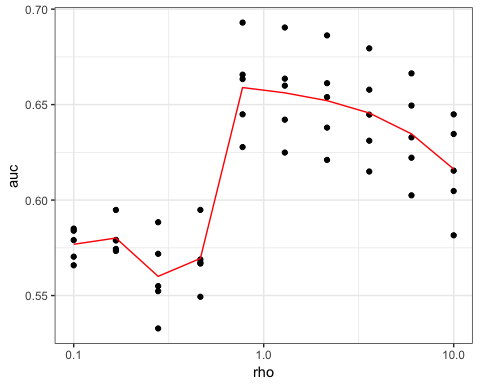``````library(dplyr)
df %>% group_by(rho) %>% summarise(mean_auc = mean(auc)) %>%
mutate(max = ifelse(max(mean_auc) == mean_auc, "***", "."))
#> # A tibble: 10 x 3
#>       rho mean_auc max
#>     <dbl>    <dbl> <chr>
#>  1  0.100    0.577 .
#>  2  0.167    0.580 .
#>  3  0.278    0.560 .
#>  4  0.464    0.569 .
#>  5  0.774    0.659 ***
#>  6  1.29     0.656 .
#>  7  2.15     0.652 .
#>  8  3.59     0.646 .
#>  9  5.99     0.635 .
#> 10 10.0      0.616 .``````

Optimal `rho` value that has the best performance to detect the anomalies is 0.774.

### 3.5 Anomaly Detection

We have obtained optimal hyperparameter `rho.` Next, let us investigate optimal threshold value for anomaly scores. We measure the performance of the anomaly detection by F-measure.

``````optimal_rho <- 0.774
fit <- sGMRFmix(train_data, K = 7, rho = optimal_rho, verbose = FALSE)

threshold_candidates <- 10^seq(-1, 1, length.out = 100)
df <- data.frame()
for (i in seq_len(n_split)) {
anomaly_score <- compute_anomaly_score(fit, split_test_data[[i]])
f_measure <- double(length(threshold_candidates))
for (j in seq_along(threshold_candidates)) {
f1 <- f1Score(unlist(split_test_labels[[i]]), unlist(anomaly_score),
cutoff = threshold_candidates[j])
f_measure[j] <- f1
}
df <- rbind(df, data.frame(cutoff = threshold_candidates, f_measure = f_measure))
}``````
``````ggplot(df, aes(cutoff, f_measure)) + geom_point() +
stat_summary(fun.y = mean, geom = "line", color = "red") + scale_x_log10()``````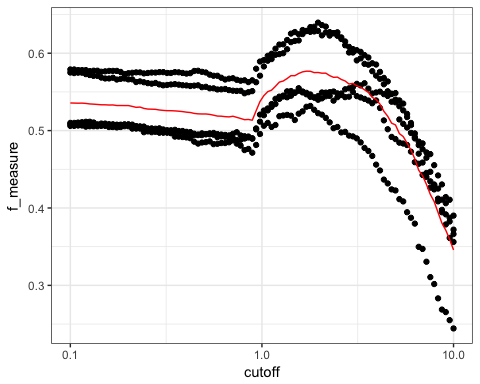``````df %>% group_by(cutoff) %>%
summarise(mean_f_measure = mean(f_measure)) %>%
filter(mean_f_measure == max(mean_f_measure))
#> # A tibble: 1 x 2
#>   cutoff mean_f_measure
#>    <dbl>          <dbl>
#> 1   1.71          0.577``````

We found optimal threshold is 1.71.

We can use the value for anomaly detection.

``````anomaly_scores <- compute_anomaly_score(fit, test_data)
is_anomaly <- anomaly_scores > 1.71
plot_multivariate_data(test_data, label = is_anomaly)``````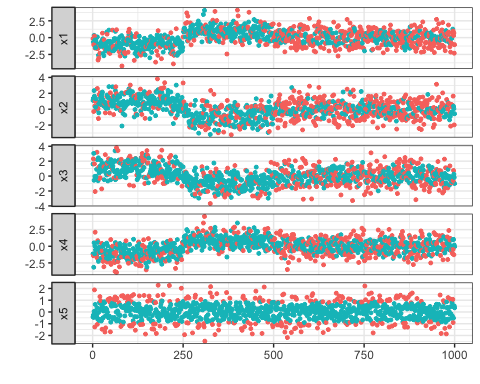### 3.6 Moving Average of Anomaly Scores

In the above example, you might think the F-measure is low. There is another way if you are interested in whether anomalies occur densely rather than individual one.

You can calculate moving average (or called rolling mean) for the anomaly scores by passing window size.

``````window_size <- 20

df <- data.frame()
for (i in seq_len(n_split)) {
anomaly_score <- compute_anomaly_score(fit, split_test_data[[i]], window_size)
f_measure <- double(length(threshold_candidates))
for (j in seq_along(threshold_candidates)) {
f1 <- f1Score(unlist(split_test_labels[[i]]), unlist(anomaly_score),
cutoff = threshold_candidates[j])
f_measure[j] <- f1
}
df <- rbind(df, data.frame(cutoff = threshold_candidates, f_measure = f_measure))
}``````
``````ggplot(df, aes(cutoff, f_measure)) + geom_point() +
stat_summary(fun.y = mean, geom = "line", color = "red") + scale_x_log10()``````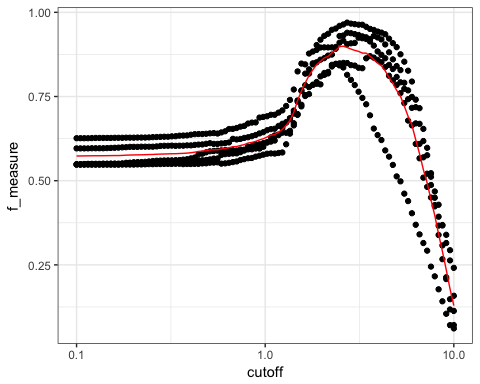``````df %>% group_by(cutoff) %>%
summarise(mean_f_measure = mean(f_measure)) %>%
filter(mean_f_measure == max(mean_f_measure))
#> # A tibble: 1 x 2
#>   cutoff mean_f_measure
#>    <dbl>          <dbl>
#> 1   2.60          0.899``````
``````anomaly_scores <- compute_anomaly_score(fit, test_data, window_size)
is_anomaly <- anomaly_scores > 2.60
plot_multivariate_data(test_data, label = is_anomaly)``````